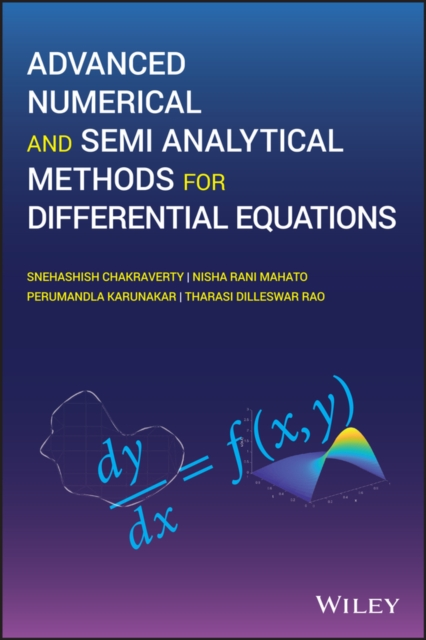# Advanced Numerical and Semi-Analytical Methods for Differential Equations Snehashish Chakraverty ; Nisha Mahato ; Perumandla Karunakar ; Tharasi Dilleswar Rao

Examines numerical and semi-analytical methods for differential equations that can be used for solving practical ODEs and PDEs This student-friendly book deals with various approaches for solving differential equations numerically or semi-analytically depending on the type of equations and offers simple example problems to help readers along. Featuring both traditional and recent methods, Advanced Numerical and Semi Analytical Methods for Differential Equations begins with a review of basic numerical methods. It then looks at Laplace, Fourier, and weighted residual methods for solving differential equations. A new challenging method of Boundary Characteristics Orthogonal Polynomials (BCOPs) is introduced next. The book then discusses Finite Difference Method (FDM), Finite Element Method (FEM), Finite Volume Method (FVM), and Boundary Element Method (BEM). Following that, analytical/semi analytic methods like Akbari Ganji's Method (AGM) and Exp-function are used to solve nonlinear differential equations. Nonlinear differential equations using semi-analytical methods are also addressed, namely Adomian Decomposition Method (ADM), Homotopy Perturbation Method (HPM), Variational Iteration Method (VIM), and Homotopy Analysis Method (HAM). Other topics covered include: emerging areas of research related to the solution of differential equations based on differential quadrature and wavelet approach; combined and hybrid methods for solving differential equations; as well as an overview of fractal differential equations. Further, uncertainty in term of intervals and fuzzy numbers have also been included, along with the interval finite element method. This book: Discusses various methods for solving linear and nonlinear ODEs and PDEs Covers basic numerical techniques for solving differential equations along with various discretization methods Investigates nonlinear differential equations using semi-analytical methods Examines differential equations in an uncertain environment Includes a new scenario in which uncertainty (in term of intervals and fuzzy numbers) has been included in differential equations Contains solved example problems, as well as some unsolved problems for self-validation of the topics covered Advanced Numerical and Semi Analytical Methods for Differential Equations is an excellent text for graduate as well as post graduate students and researchers studying various methods for solving differential equations, numerically and semi-analytically.
€110,00

### Specificaties

ISBN
9781119423423
Uitgever
John Wiley and Sons Ltd
Druk
1e
Verschenen
29-03-2019
Taal
Engels
256 pp.
Bindwijze
Hardcover
Genre
Wetenschap

### Koop uw boeken bij Athenaeum

• Gratis verzending vanaf € 20,- (in de algemene webwinkel, binnen Nederland).
• Bestellen zonder registratie of login.
• Vertrouwde service, veilige afhandeling.